# OzGrid

Math functions excel formulae sheet

Category: [Excel]  Demo Available

Math functions Excel Formulas Cheat Sheet

 =SUM Add the total of a series of numbers.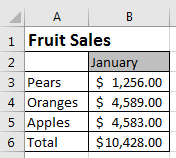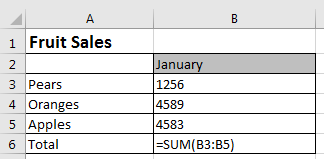=AVERAGE Calculates the average of a series of numbers.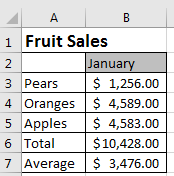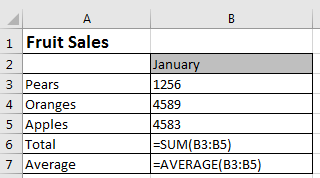=MEDIAN Returns the median average number of a series.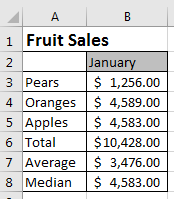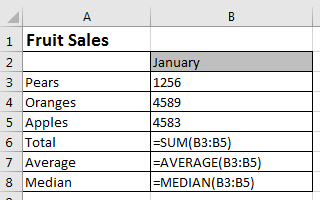=SUMPRODUCT Calculates the weighted average, very useful for financial analysis.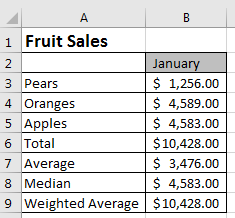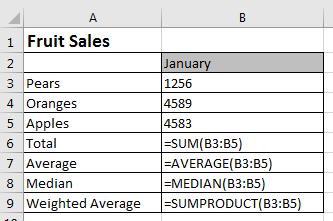=PRODUCT Multiplies all of a series of numbers.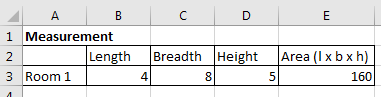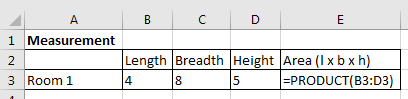=ROUNDDOWN Rounds a number to the specified number of digits. =ROUNDDOWN(number,num_digits)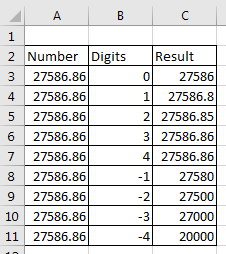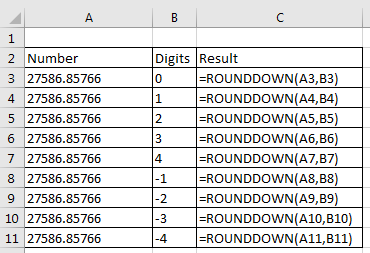=ROUNDUP The formula rounds a number to the specific number of digits.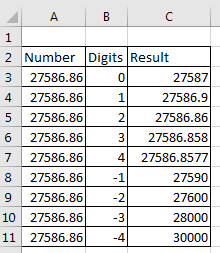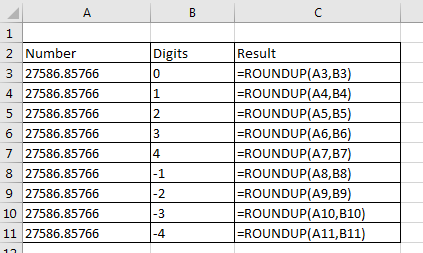=ABS Returns the absolute value of a number. Negative numbers are converted to positive numbers and positive numbers stay the same.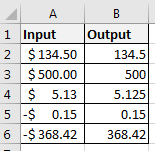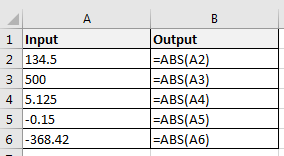=PI Returns the value of pi, accurate to 15 digits.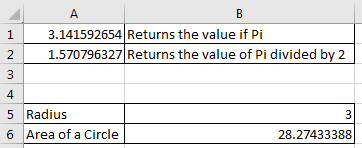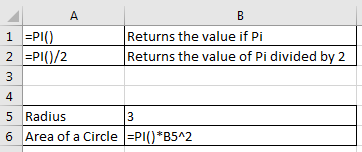=SUMIF Sum values in a range that are specified by a condition.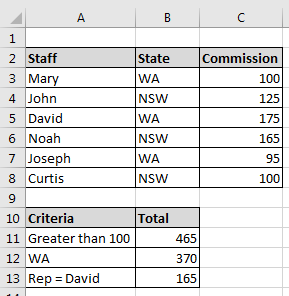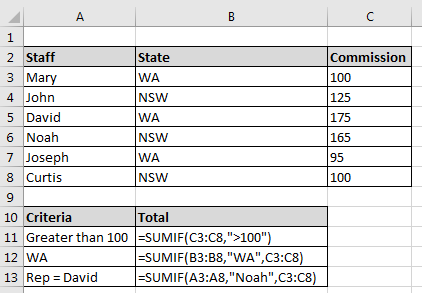Free Training Course: Lesson 1 - Excel Fundamentals

Microsoft ® and Microsoft Excel ® are registered trademarks of Microsoft Corporation. OzGrid is in no way associated with Microsoft.

Click here to visit our Free 24/7 Excel/VBA Help Forum where there are thousands of posts you can get information from, or you can join the Forum and post your own questions.

### Gallery

stars (0 Reviews)